# Data Science. Probability distributions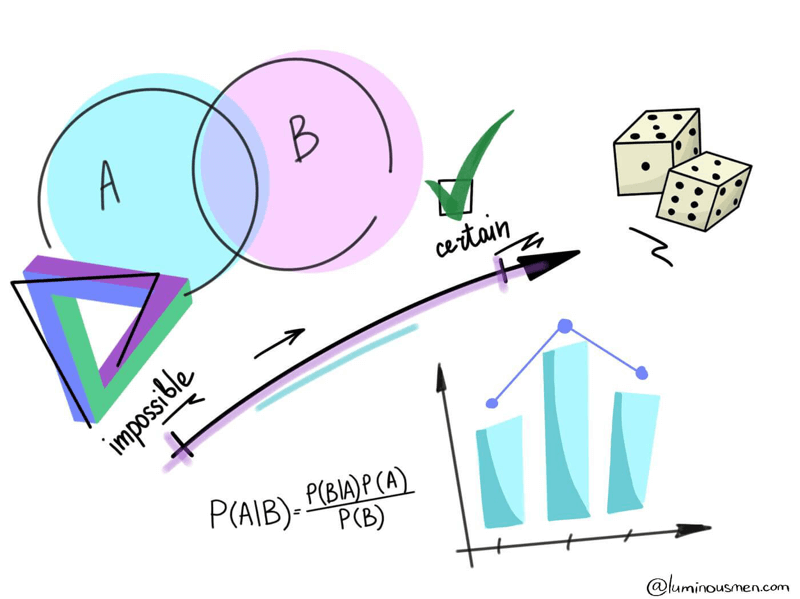There are a lot of engineers who have never been involved in the field of statistics or data science. But in order to build a data science pipelines or rewrite produced code by data scientists to an adequate, easily maintained code many nuances and misunderstandings arise from the engineering side. For those Data/ML engineers and novice data scientists, I make this series of posts. I'll try to explain some basic approaches in plain English and, based on it, explain some of the Data Science basic concepts.

The whole series:

There are many distributions, but here, we will be talking about the most common and used ones. But first, we need to understand probability functions for continuous random variables.

## Probability Density Function(pdf)

Let's consider an experiment in which the probability of events is as follows. The probability of getting numbers 1,2,3,4 is 1/10, 2/10, 3/10, 4/10 respectively. It would be more convenient for us if we had an equation for that experiment that would give us those values based on the probability of events. For example, the equation for this experiment can be set to f(x)=x/10, where x=1,2,3,4. This equation (or function) is called probability distribution function. Although some authors also call it a probability function, a frequency function or a probability mass function. It tells us that a random variable x is likely to appear.

## Cumulative Distribution Function(cdf)

The cumulative distribution function provides an integral picture of the probability distribution. As the name cumulative suggests, it is simply the probability that a variable will take a value less than or equal to a particular value. In the example above given x=3, the cdf tells us the sum probability of all random variables from 1 to 3.

## Continuous distributions

In this section, as the title suggests, we investigate probabilistic distributions of continuous random variables, i.e. random variables whose support contains an infinite range of possible outcomes.

### Uniform Distribution

A uniform distribution is a type of distribution of probabilities where all outcomes are equally likely; each variable has the same probability that it will be the outcome. A deck of cards has within its uniform distributions because the probability that a heart, club, diamond, or spade is pulled is the same. The coin also has a uniform distribution because the probability of either the head or the tail in the coin toss is the same.

from scipy.stats import uniform
import matplotlib.pyplot as plt
import numpy as np

def uniform() -> None:
fig, ax = plt.subplots(1, 1, figsize=(15,15))
# calculate a few first moments
mean, var, skew, kurt = uniform.stats(moments='mvsk')
# display the probability density function (pdf)
x = np.linspace(uniform.ppf(0.01), uniform.ppf(0.99), 100)
ax.plot(x, uniform.pdf(x),
'r-', lw=5, alpha=0.6, label='uniform pdf')
ax.plot(x, uniform.cdf(x),
'b-', lw=5, alpha=0.6, label='uniform cdf')
# Check accuracy of cdf and ppf
vals = uniform.ppf([0.001, 0.5, 0.999])
np.allclose([0.001, 0.5, 0.999], uniform.cdf(vals))

# generate random numbers
r = uniform.rvs(size=1000)
# and compare the histogram
ax.hist(r, normed=True, histtype='stepfilled', alpha=0.2)
ax.legend(loc='best', frameon=False)
plt.show()

uniform()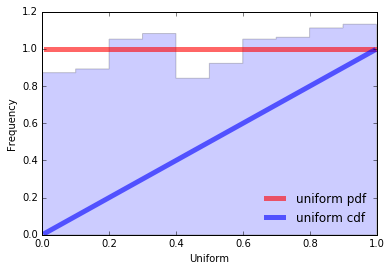More formally this is the distribution of a random variable that can take any value in the interval (a, b), and the probability of being in any segment inside (a, b) is proportional to the length of the segment and does not depend on its position, and the probability of values outside the interval (a, b) is equal to 0.

So, a continuous random variable x has a uniform distribution, denoted U(a, b), if its probability density function is:

$$f(x)=\dfrac{1}{b-a}$$

### Normal Distribution

from scipy.stats import norm
import matplotlib.pyplot as plt
import numpy as np

def normal() -> None:
fig, ax = plt.subplots(1, 1)
# calculate a few first moments
mean, var, skew, kurt = norm.stats(moments='mvsk')
# display the probability density function (pdf)
x = np.linspace(norm.ppf(0.01),  norm.ppf(0.99), 100)
ax.plot(x, norm.pdf(x),
'r-', lw=5, alpha=0.6, label='norm pdf')
ax.plot(x, norm.cdf(x),
'b-', lw=5, alpha=0.6, label='norm cdf')
# check accuracy of cdf and ppf
vals = norm.ppf([0.001, 0.5, 0.999])
np.allclose([0.001, 0.5, 0.999], norm.cdf(vals))

# generate random numbers:
r = norm.rvs(size=1000)
# and compare the histogram
ax.hist(r, normed=True, histtype='stepfilled', alpha=0.2)
ax.legend(loc='best', frameon=False)
plt.show()

normal()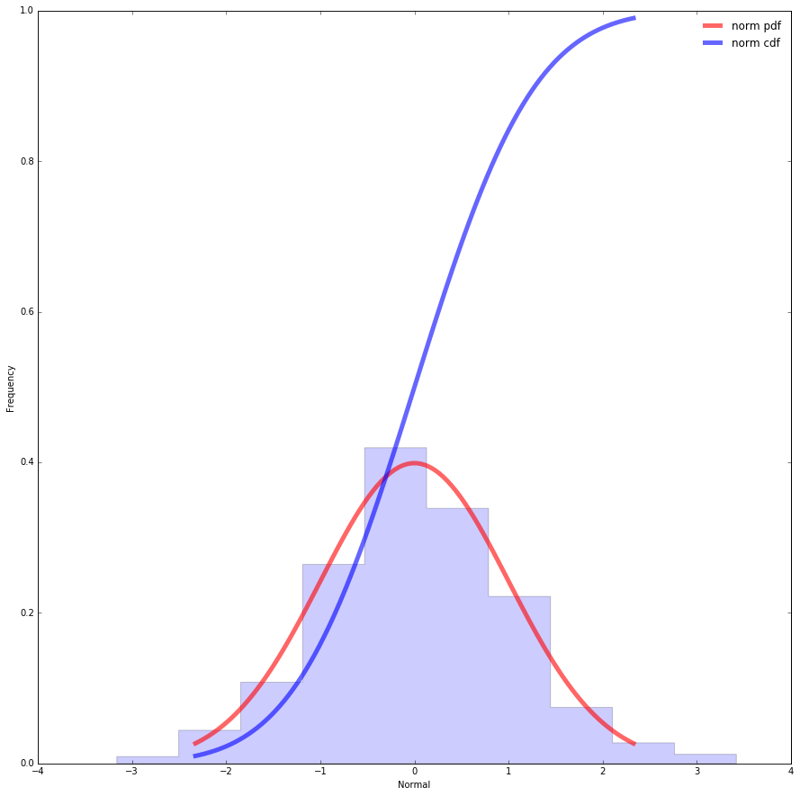At the heart of the statistics lies the normal distribution, known to millions of people as a bell-shaped curve. It is a two-parameter family of curves that represent plots of probability density functions:

$$f(x) = \frac{1}{\sigma \sqrt{2\pi}} \exp\left(−\frac{(x−\mu)^2}{2\sigma^2}\right)$$

It looks a little scary, but we'll get it all figured out soon enough. The normal distribution density function has two mathematical constants:

• π — the ratio of the circle to its diameter is about 3,142;
• e — the base of the natural logarithm is about 2.718;

And two parameters that set the shape of a particular curve:

• µ is a mathematical expectation or mean. It shows that data near the mean are more frequent in occurrence than data far from the mean.
• $σ^2$ — variance, will also be discussed in the next posts;

And, of course, the variable x itself for which the function value is calculated, i.e. the probability density.

The constants, of course, don't change. But parameters are what give the final shape to a particular normal distribution.

So, the specific form of the normal distribution depends on 2 parameters: the expectation (µ) and variance ($σ^2$). Briefly denoted by $N(m, σ^2)$. The parameter µ (expectation) determines the distribution center, which corresponds to the maximum height of the graph. The variance $σ^2$ characterizes the range of variation, that is, the “spread” of the data.

Another interesting detail of this distribution is when we calculate the standard deviation we find that:

• about 68% of values are within 1 standard deviation of the mean
• about 95% of values are within 2 standard deviations of the mean
• about 99.7% of values are within 3 standard deviations of the mean

Why is this distribution so popular?

The importance of normal distributions is primarily due to the fact that the distributions of many natural phenomena are at least roughly normally distributed. One of the first applications of normal distributions was the analysis of errors of measurements made in astronomical observations, errors caused by imperfect instruments and imperfect observers. Galileo noted in the seventeenth century that these errors were symmetrical and that small errors occurred more frequently than large ones. This led to several hypothetical distributions of errors, but it was not until the early 19th century that it was discovered that these errors followed a normal distribution. Independently, the mathematicians Adrain in 1808 and Gauss in 1809 developed a formula for the normal distribution and showed that errors are aligned with this distribution.

## Discrete distributions

### Bernoulli distribution(binomial distribution)

from scipy.stats import bernoulli
import seaborn as sb

def bernoulli_dist(): -> None:
data_bern = bernoulli.rvs(size=1000,p=0.6)
ax = sb.distplot(
data_bern,
kde=True,
color='b',
hist_kws={'alpha':1},
kde_kws={'color': 'r', 'lw': 3, 'label': 'KDE'})
ax.set(xlabel='Bernouli', ylabel='Frequency')

bernoulli_dist()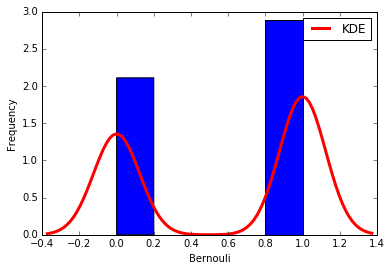Not all phenomena are measured on a quantitative scale of type 1, 2, 3 ... 100500... Not always a phenomenon can take on an infinite or a large number of different states. For example, a person’s sex can be either a man or a woman. The shooter either hits the target or misses. You can vote either "for" or "against", etc. Other words reflect the state of an alternative feature (the event did not come). The upcoming event (positive outcome) is also called "success". Such phenomena can also be massive and random. Therefore, they can be measured and make statistically valid conclusions.

Experiments with such data are called the Bernoulli scheme, in honor of the famous Swiss mathematician, who found that with a large number of tests, the ratio of positive outcomes to the total number of tests converges to the probability of the occurrence of this event.

$$f(x)=\dbinom{n}{x} p^x (1-p)^{n-x}$$

• n — the number of experiments in the series;
• x — a random variable (the number of occurrences of the event);
• $p^x$ — the probability that event happens exactly x times;
• q = 1 - p (the probability that the event does not appear in the test)

### Poisson Distribution

from scipy.stats import poisson
import seaborn as sb
import numpy as np
import matplotlib.pyplot as plt

def poisson_dist(): -> None
plt.figure(figsize=(15,15))
data_binom = poisson.rvs(mu=4, size=10000)

ax = sb.distplot(data_binom, kde=True, color='b',
bins=np.arange(data_binom.min(), data_binom.max() + 1),
kde_kws={'color': 'r', 'lw': 3, 'label': 'KDE'})
ax.set(xlabel='Poisson', ylabel='Frequency')

poisson_dist()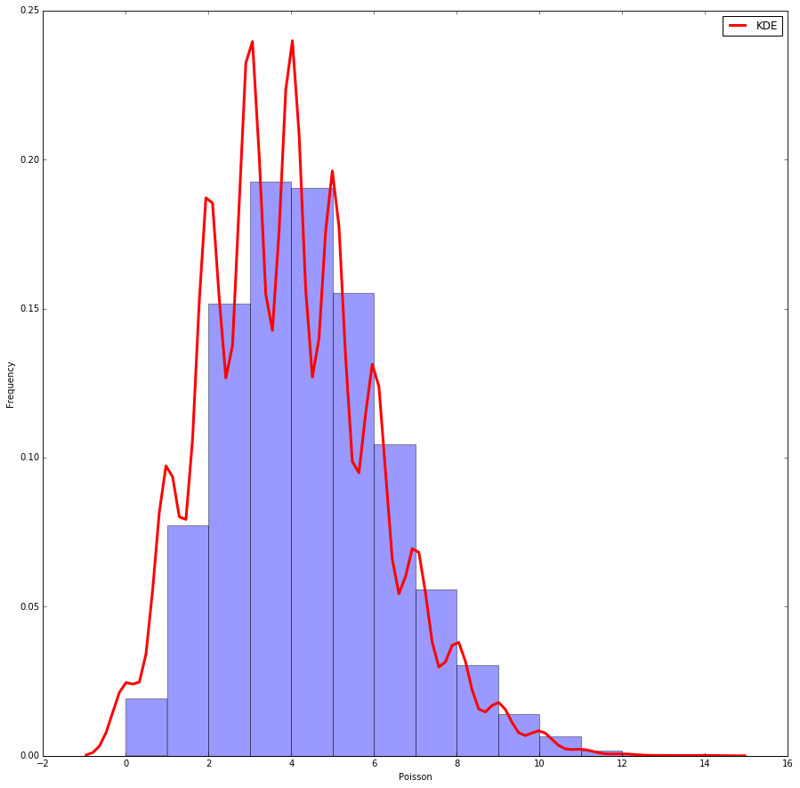The Poisson distribution is obtained as a limiting case of the Bernoulli distribution, if we push p to zero and n to infinity, but so that their product remains constant: n*p = a. Formally, such a transition leads to the formula

$$f(x) = \frac{{e^{ - \lambda } \lambda ^x }}{{x!}}$$

• x is a random variable (the number of occurrences of event A);
• $\lambda$ is the event rate(average number of events in an interval) also called the rate parameter. It is also equal to mean and variance.

Poisson's distribution is subject to many random variables that arise in scientific and practical life: equipment breaks, the duration of maintenance work performed by working staff, printing errors, the number of goals scored by the football team.

## Conclusion

There are many theoretical distributions: Normal, Poisson, Student, Fisher, Binomial and others. Each of them is intended for analysis of data of different origin and has certain characteristics. In practice these distributions are used as some template for analysis of real data of similar type. In other words, they try to impose the structure of the chosen theoretical distribution on real data, thus calculating the characteristics that are of interest to the analyst.

More precisely, theoretical distributions are probabilistic-statistical models whose properties are used to analyze empirical data. Something like that is done. Data are collected and compared to any known theoretical distributions. If there are similarities, the properties of a theoretical model are transmitted to the empirical data with the corresponding conclusions. This approach is the basis for classical methods related to the hypotheses testing (calculation of confidence intervals, comparison of mean values, verification of parameters' significance, etc.).

If the available data do not correspond to any known theoretical distribution (which usually happens in practice, but for some reason, nobody cares), it is not recommended to use the selected template (probabilistic-statistical model). Illegal use of parametric distributions leads to the situation when an analyst searches for keys not where he has lost them, but under a street lamp pole where it is light. To solve this problem, there are other approaches related to using non-parametric statistics.Buy me a coffee